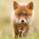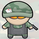1144 görüntülenme
grid based on the cumulative average price.

on a sidenote: looks like im baned from chat for a week.
```study("[RS]CAP Grid", overlay=true)

c = cum(close)/(1+n)
h = cum(high)/(1+n)
l = cum(low)/(1+n)

factor = h - l

plot(c, color=black, linewidth=2)
plot(h, color=black, linewidth=2)
plot(h + factor, color=black, linewidth=2)
plot(h + factor * 2, color=black, linewidth=2)
plot(h + factor * 3, color=black, linewidth=2)
plot(h + factor * 4, color=black, linewidth=2)
plot(h + factor * 5, color=black, linewidth=2)

plot(l, color=black, linewidth=2)
plot(l - factor, color=black, linewidth=2)
plot(l - factor * 2, color=black, linewidth=2)
plot(l - factor * 3, color=black, linewidth=2)
plot(l - factor * 4, color=black, linewidth=2)
plot(l - factor * 5, color=black, linewidth=2)
```

## YorumlarInteresting approach! Have you tried using as "factor multipliers" fibonacci/padovan progressions..? ;)
Cevap Gönderxel_arjona
possibly, likely unpublished, just a matter of changing the multipliers.
the padovan sequence looks interesting, 1st time i heard of it :p
Cevap GönderRicardoSantos
When you help me to learn pine when writing my "Fractal Bands", we wrote the algorithm to calculate mathematically both of this progressions in pine. (recursive innecesary but fur fun! ) :D
// N Root Function
nroot(index,number) =>
cond_r = index == 0 or number == 0 or number < 0
If_True = 0
If_False = (exp((1/index) * log(number)))
iff(cond_r, If_True, If_False)
//Algebraic Constants for general calculations.
_phi = (1+sqrt(5))/2 // Phi Number (Fibonacci Seq.)
_pn = (nroot(3,(108 + 12*sqrt(69))) + nroot(3,(108 - 12*sqrt(69))))/6 // Plastic Number (Podovan Seq.)
Cevap Gönderxel_arjona
aw i see, i remember the fib number function :p
Cevap GönderRicardo, interesting indicator.
I coded an EMA with ATR bands that serves a similar purpose.

Can I pm you with a request?
I'm always impressed by your coding skills.
Cevap GönderI'm curious. Why does the distance between central lines are shorter than others?
Cevap Gönderalex.a
they are the respective lines for the cumulative average of high close and low.
Cevap GönderRicardoSantos
I should I interpret that, if the price was in that range, usually the distance between H and L were shorter???
Cevap Gönderalex.a
in my opinion it would mean its at its normal price, end/start of cycle
Cevap Gönder